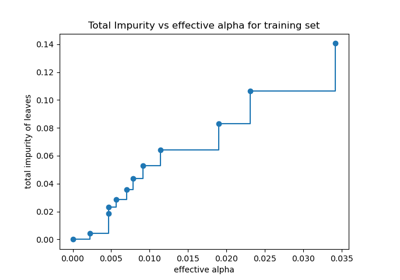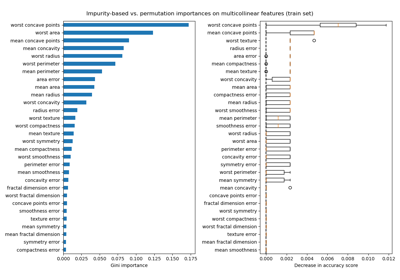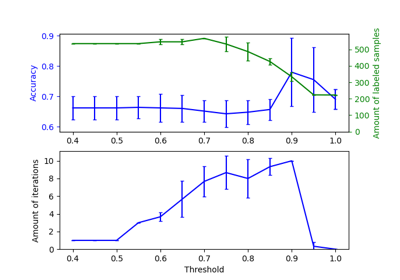# sklearn.datasets.load_breast_cancer¶

Load and return the breast cancer wisconsin dataset (classification).

The breast cancer dataset is a classic and very easy binary classification dataset.

 Classes 2 Samples per class 212(M),357(B) Samples total 569 Dimensionality 30 Features real, positive

The copy of UCI ML Breast Cancer Wisconsin (Diagnostic) dataset is downloaded from: https://goo.gl/U2Uwz2

Read more in the User Guide.

Parameters:
return_X_ybool, default=False

If True, returns (data, target) instead of a Bunch object. See below for more information about the data and target object.

New in version 0.18.

as_framebool, default=False

If True, the data is a pandas DataFrame including columns with appropriate dtypes (numeric). The target is a pandas DataFrame or Series depending on the number of target columns. If return_X_y is True, then (data, target) will be pandas DataFrames or Series as described below.

New in version 0.23.

Returns:
dataBunch

Dictionary-like object, with the following attributes.

data{ndarray, dataframe} of shape (569, 30)

The data matrix. If as_frame=True, data will be a pandas DataFrame.

target{ndarray, Series} of shape (569,)

The classification target. If as_frame=True, target will be a pandas Series.

feature_nameslist

The names of the dataset columns.

target_nameslist

The names of target classes.

frameDataFrame of shape (569, 31)

Only present when as_frame=True. DataFrame with data and target.

New in version 0.23.

DESCRstr

The full description of the dataset.

filenamestr

The path to the location of the data.

New in version 0.20.

(data, target)tuple if return_X_y is True

A tuple of two ndarrays by default. The first contains a 2D ndarray of shape (569, 30) with each row representing one sample and each column representing the features. The second ndarray of shape (569,) contains the target samples. If as_frame=True, both arrays are pandas objects, i.e. X a dataframe and y a series.

New in version 0.18.

Examples

Let’s say you are interested in the samples 10, 50, and 85, and want to know their class name.

>>> from sklearn.datasets import load_breast_cancer
>>> data.target[[10, 50, 85]]
array([0, 1, 0])
>>> list(data.target_names)
['malignant', 'benign']


## Examples using sklearn.datasets.load_breast_cancer¶Post pruning decision trees with cost complexity pruning

Post pruning decision trees with cost complexity pruningPermutation Importance with Multicollinear or Correlated Features

Permutation Importance with Multicollinear or Correlated FeaturesEffect of varying threshold for self-training

Effect of varying threshold for self-training